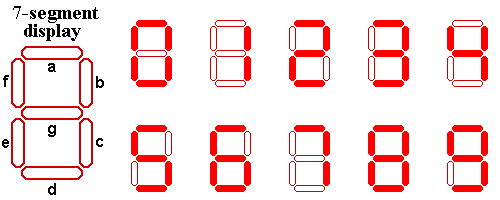# Seven-Segment Display | Practice problem Hackerearth solution

Problem:-

You all must have seen a seven segment display.If not here it is:Alice got a number written in seven segment format where each segment was creatted used a matchstick.

Example: If Alice gets a number 123 so basically Alice used 12 matchsticks for this number.

Alice is wondering what is the numerically largest value that she can generate by using at most the matchsticks that she currently possess.Help Alice out by telling her that number.

Input Format:

First line contains (test cases).

Next lines contain a Number N.

Output Format:

Print the largest possible number numerically that can be generated using at max that many number of matchsticks which was used to generate N.

Constraints:

$1\le T\le 100$

$1\le length\left(N\right)\le 100$

Sample Input
`210`
Sample Output
`1111`
Time Limit: 1
Memory Limit: 256
Source Limit:
Explanation

If you have as your number that means you have match sticks.You can generate 1 using this.

If you have as your number that means you have match sticks.You can generate 111 using this.

Code:-

Here I am going to give you two solution first one is on the basis of C language and second one is on the basis of c++ language which you can submit in c++14 and c++17 also

Solution 1 ( C language):-

#include<string.h>
int main()
{
int t;
scanf(“%d”,&t);
while(t–)
{
int a={6,2,5,5,4,5,6,3,7,6,},i,sum=0;
char n;
scanf(“%s”,n);
for(i=0;i<strlen(n);i++)
sum+= a[(n[i]-48)];
if(sum%2==1)
printf(“7”);
else
printf(“1”);
for(i=1;i<sum/2;i++)
printf(“1”);
printf(“n”);
}
return 0;
}

Solution 2 ( C++ language):-

This solution is based on the c++ language and you can submit ib c++14 and c++17 also.
#include <bits/stdc++.h>
using namespace std;
int main()
{
int T;
cin >> T;
string str;
// taking string input
int t =0;
while( t != T){
cin >> str;
t++;
// array with size
int arr = {6,2,5,5,4,5,6,3,7,6};
int m = str.size();
int a[m];
for(int i = 0; i<m; i++){
a[i] = str[i] ‘0’;
}
int sum = 0;
for( int i = 0; i<m; i++){
sum += arr[a[i]];
}
while(sum != 0){
if(sum%2 != 0){
sum -= 3;
cout << 7;
}
else{
sum -= 2;
cout << 1;
}
}
cout << endl;
}
}

Recommended Post:-